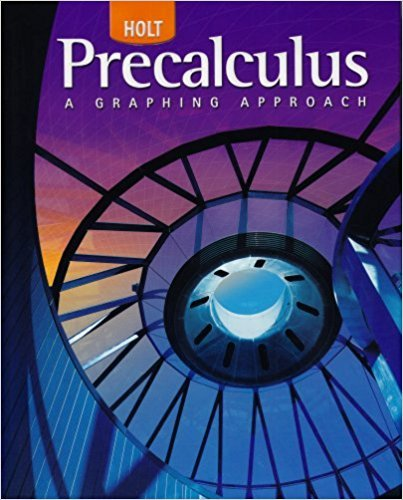×
×

# Solutions for Chapter 9.2: Thomas W. Hungerford## Full solutions for Precalculus | 1st Edition

ISBN: 9780030416477Solutions for Chapter 9.2: Thomas W. Hungerford

Solutions for Chapter 9.2
4 5 0 249 Reviews
18
2
##### ISBN: 9780030416477

Precalculus was written by and is associated to the ISBN: 9780030416477. This textbook survival guide was created for the textbook: Precalculus, edition: 1. Chapter 9.2: Thomas W. Hungerford includes 66 full step-by-step solutions. Since 66 problems in chapter 9.2: Thomas W. Hungerford have been answered, more than 26070 students have viewed full step-by-step solutions from this chapter. This expansive textbook survival guide covers the following chapters and their solutions.

Key Calculus Terms and definitions covered in this textbook
• Cardioid

A limaçon whose polar equation is r = a ± a sin ?, or r = a ± a cos ?, where a > 0.

• Directrix of a parabola, ellipse, or hyperbola

A line used to determine the conic

• Exponential decay function

Decay modeled by ƒ(x) = a ? bx, a > 0 with 0 < b < 1.

• Future value of an annuity

The net amount of money returned from an annuity.

• Hyperboloid of revolution

A surface generated by rotating a hyperbola about its transverse axis, p. 607.

• Hypotenuse

Side opposite the right angle in a right triangle.

• Logarithmic re-expression of data

Transformation of a data set involving the natural logarithm: exponential regression, natural logarithmic regression, power regression

• Matrix element

Any of the real numbers in a matrix

• Multiplicative inverse of a real number

The reciprocal of b, or 1/b, b Z 0

• Parameter

See Parametric equations.

• Polynomial function

A function in which ƒ(x)is a polynomial in x, p. 158.

• Reflexive property of equality

a = a

• Row echelon form

A matrix in which rows consisting of all 0’s occur only at the bottom of the matrix, the first nonzero entry in any row with nonzero entries is 1, and the leading 1’s move to the right as we move down the rows.

• RRAM

A Riemann sum approximation of the area under a curve ƒ(x) from x = a to x = b using x1 as the right-hand end point of each subinterval.

• Semimajor axis

The distance from the center to a vertex of an ellipse.

• Standard form of a polynomial function

ƒ(x) = an x n + an-1x n-1 + Á + a1x + a0

• Stemplot (or stem-and-leaf plot)

An arrangement of a numerical data set into a specific tabular format.

• Symmetric difference quotient of ƒ at a

ƒ(x + h) - ƒ(x - h) 2h

• Third quartile

See Quartile.

• Triangular number

A number that is a sum of the arithmetic series 1 + 2 + 3 + ... + n for some natural number n.

×